# Extract data from invoices with Mindee's API using Python

In this tutorial, you will learn how to extract data from invoices (pdfs or images) using python. This can help you automatically process invoices in any accounting software.

The final script can be used within any python middleware application or REST API serving your frontend interfaces.

In the end of this tutorial, you will learn how to transform a pdf into an image file, and how to highlight features on it to make human validation easier.

The final result looks like this: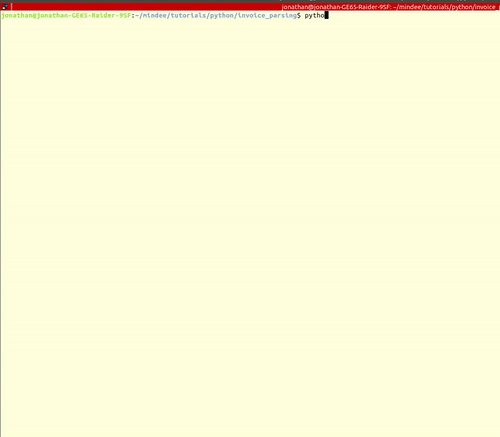## API Prerequisites

2. An invoice, look into your mailbox or check out on google image

## Setup the project

Create an empty directory wherever you want in your laptop, we’ll call ours “python_invoice’, and create a ‘main.py’ file in it.

Open a terminal and go to the directory you just created. In this tutorial we’ll use the python library “requests” to call the API. If it’s not installed in your env, you can install by running:

``pip install requests``

Make sure you have an invoice somewhere on your laptop, and you know the path.

That’s it for the setup, let’s call the API.

## Call the Mindee Invoice API

Login onto the [Mindee dashboardt](https://platform.mindee.net/) and enter the Invoice API environment by clicking the following card.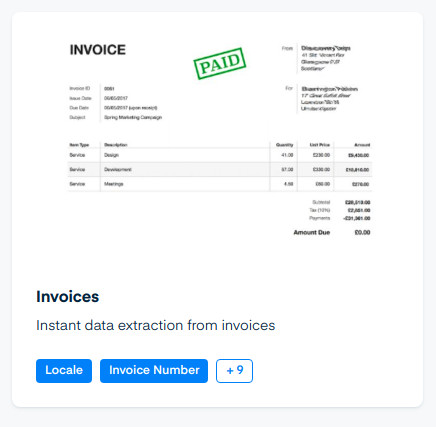If you don’t have an API token yet for this API, go to the “credentials” section and create a new API Key.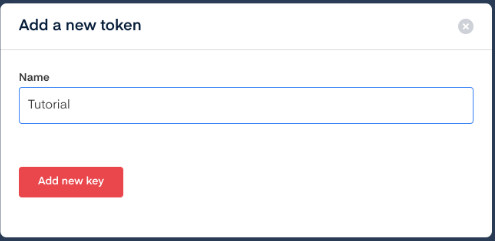Click on the documentation section in the navbar, and then click on the Python link on the sample code area. Copy the code.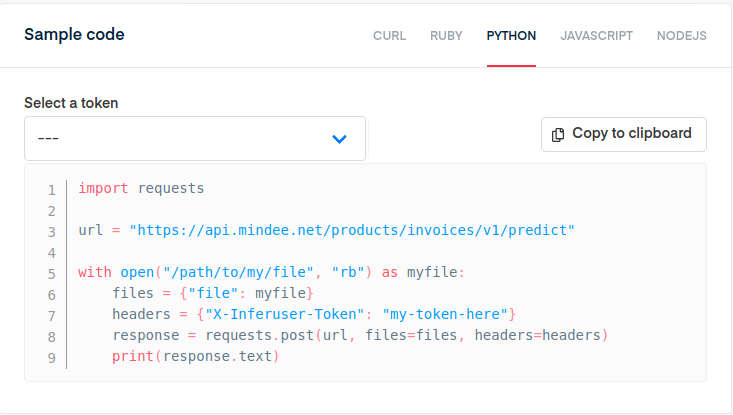Open the “main.py” file you created a few minutes ago, and paste your sample code.  The path to your invoice will be placed in the variable "filepath" since we call it several times. Your main.py file should look like:

``````import requests

filepath = "/path/to/your/file"
url = "https://api.mindee.net/products/invoices/v1/predict"

with open(filepath, "rb") as myfile:
files = {"file": myfile}
print(response.text)
``````

Replace the “path/to/my/file” placeholder in the code with the path of the invoice pdf or image you want to parse. Replace the “my-token-here” with the API token you created previously in the platform.

You can get back to your console and run the script

``python main.py``

You should see a json object appearing in your console. Now let’s parse this json to get the data we need.

## Parse the result

The API returns a list of different fields in the invoice (total amount, taxes, date …). You can find this list in the “documentation” section in Mindee platform.

Now that we coded the API request, we’ll use the json object and get a few extracted features.

First, let’s create a parsing function that returns the invoice total amount, the invoice date of issuance, and the taxes. We’ll focus only on those three features for the tutorial but you can find more in the documentation. The features are inside the prediction object in the json response.

Note: As you can send a multi-page pdf to the API, the predictions object is an array, with a prediction object for each page.

In our case, as we are sending a single page pdf, the predictions array contains only one object. As the taxes object returned is an array with different tax objects, we are just going to iterate over the different taxes and concatenate values in a string so we can easily display it.

``````def get_features(json_response):
parsed_data = {}
prediction = json_response["predictions"]

# get features values
parsed_data["total_incl"] = prediction["total_incl"]["amount"]
parsed_data["date"] = prediction["invoice_date"]["iso"]
parsed_data["taxes"] = []
for tax in prediction["taxes"]:
parsed_data["taxes"].append(str(tax["amount"])+ " "+str(tax["rate"])+"%")

return parsed_data``````

Now that our function is ready, we’ll just print the result in the console and add a simple check to ensure the API call was well performed and we can access the result. You can replace the main.py script to this:

``````import requests

def get_features(json_response):
parsed_data = {}
prediction = json_response["predictions"]

# get features values
parsed_data["total_incl"] = prediction["total_incl"]["amount"]
parsed_data["date"] = prediction["invoice_date"]["iso"]
parsed_data["taxes"] = []
for tax in prediction["taxes"]:
parsed_data["taxes"].append(str(tax["amount"])+ " "+str(tax["rate"])+"%")

return parsed_data

filepath = "/path/to/your/file"
url = "https://api.mindee.net/products/invoices/v1/predict"

with open(filepath, "rb") as myfile:
files = {"file": myfile}

if response.status_code != 200:
print("Request error")
else:
json_response = response.json()
features = get_features(json_response)
print("Date:", features["date"])
print("Taxes: ", ' - '.join(features["taxes"]))
print("Total amount including taxes:", features["total_incl"])``````

Run the script and check out the results on your image.

Now we are going to add a piece of code that will highlight the features extracted on the pdf. It can help you or your users to very quickly validate the data extraction.

## Convert pdf into an image

If you’re using a pdf, we need first to convert the pdf into an image, as it’s more convenient to use for highlighting the features on it. If your invoice is an image, you can skip this part.

To convert the pdf into a jpeg we are going to use the pdf2image python library; First install it in your environment:

``pip install pdf2image``

Important note: Dealing with pdfs is not easy and this library might not work directly because it sometimes requires some extra dependencies. Check out the github to make sure you installed it correctly.

Once installed, we are going to create an image out of our pdf:

``````from pdf2image import convert_from_path, convert_from_bytes
images = convert_from_path(filepath)``````

The images object is an array of Pillow images. Pillow is an image library for python. As we want to use openCV for highlighting the features on the image, we need to convert the pillow image into an OpenCV image. OpenCV is based on the numpy library, and to convert it we are going to use numpy directly.

If you don’t have numpy installed on your environment:

``pip install numpy``

Now let’s convert the Pillow image into an opencv format using numpy:

``````import numpy as np
from pdf2image import convert_from_path

def pdf_to_cv_image(filepath, page=0):
image = convert_from_path(filepath)[page]
return np.asarray(image)``````

## Highlight features on the image

Let’s try to highlight the features as if someone did it with a pen.

First, you’ll need to install the computer vision python library OpenCV if you don’t have it already installed in your env. To do so, run:

``pip install opencv-python``

We need to change a bit our get_features function to return coordinates for each feature, as we're using this to know where to draw the rectangles.

``````def get_features(json_response):
parsed_data = {}
coordinates = []

prediction = json_response["predictions"]

# get features values
parsed_data["total_incl"] = prediction["total_incl"]["amount"]
parsed_data["date"] = prediction["invoice_date"]["iso"]
parsed_data["taxes"] = []
for tax in prediction["taxes"]:
parsed_data["taxes"].append(str(tax["amount"])+ " "+str(tax["rate"])+"%")

# get features bounding boxes
coordinates.append(prediction["total_incl"]["segmentation"]["bounding_box"])
coordinates.append(prediction["invoice_date"]["segmentation"]["bounding_box"])

for tax in prediction["taxes"]:
coordinates.append(tax["segmentation"]["bounding_box"])

return parsed_data, coordinates``````

Once it’s done, we are going to create our highlighter function in different steps, as follows:

1. Check if the file is a pdf or an image and load it as an openCV image in both scenarios
3. Loop on each feature coordinates and draw the feature rectangle on our mask
4. Overlay the mask and original image with alpha
5. Display image to the user

Note: each coordinate returned by the API is relative (in % of the image). You'll see there is a relative to absolute conversion in the code.

Here is the code step by step:

``````def highlight_features(img_path, coordinates):
# step 1: Check if the file is a pdf or an image and load it as an openCV image in both scenarios
if img_path.endswith(".pdf"):
cv_image = pdf_to_cv_image(img_path)
else:

# step 2: create mask image
overlay = cv_image.copy()
h, w = cv_image.shape[:2]

# step 3: Loop on each feature coordinates and draw the feature rectangle on our mask
for coord in coordinates:
if len(coord):
pt1 = (int(w*coord), int(h*coord))
pt2 = (int(w*coord), int(h*coord))
cv2.rectangle(overlay, pt1, pt2, (70, 230, 244), cv2.FILLED)

# step 4: Overlay the mask and original image with alpha
final_image = cv2.addWeighted(overlay, 0.5, cv_image, 0.5, 0)

# step 5: Display image to the user
cv2.imshow("highlghted_image", cv2.resize(final_image, (600, int(600*h/w))))
cv2.waitKey(0)``````

Finally, we just have to modify a bit the code for executing the highlighting function and printing the results. Here is what the final code:

``````import requests
import cv2
import numpy as np
from pdf2image import convert_from_path

def pdf_to_cv_image(filepath, page=0):
image = convert_from_path(filepath)[page]
return np.asarray(image)

def get_features(json_response):
parsed_data = {}
coordinates = []

prediction = json_response["predictions"]

# get features values
parsed_data["total_incl"] = prediction["total_incl"]["amount"]
parsed_data["date"] = prediction["invoice_date"]["iso"]
parsed_data["taxes"] = []
for tax in prediction["taxes"]:
parsed_data["taxes"].append(str(tax["amount"])+ " "+str(tax["rate"])+"%")

# get features bounding boxes
coordinates.append(prediction["total_incl"]["segmentation"]["bounding_box"])
coordinates.append(prediction["invoice_date"]["segmentation"]["bounding_box"])

for tax in prediction["taxes"]:
coordinates.append(tax["segmentation"]["bounding_box"])

return parsed_data, coordinates

def highlight_features(img_path, coordinates):
# step 1: Check if the file is a pdf or an image and load it as an openCV image in both scenarios
if img_path.endswith(".pdf"):
cv_image = pdf_to_cv_image(img_path)
else:

# step 2: create mask image
overlay = cv_image.copy()
h, w = cv_image.shape[:2]

# step 3: Loop on each feature coordinates and draw the feature rectangle on our mask
for coord in coordinates:
if len(coord):
pt1 = (int(w*coord), int(h*coord))
pt2 = (int(w*coord), int(h*coord))
cv2.rectangle(overlay, pt1, pt2, (70, 230, 244), cv2.FILLED)

# step 4: Overlay the mask and original image with alpha
final_image = cv2.addWeighted(overlay, 0.5, cv_image, 0.5, 0)

# step 5: Display image to the user
cv2.imshow("highlghted_image", cv2.resize(final_image, (600, int(600*h/w))))
cv2.waitKey(0)

filepath = "/path/to/your/file"
url = "https://api.mindee.net/products/invoices/v1/predict"

with open(filepath, "rb") as myfile:
files = {"file": myfile}

if response.status_code != 200:
print("Request error")
else:
json_response = response.json()
features, coords = get_features(json_response)
print("Date:", features["date"])
print("Taxes: ", ' - '.join(features["taxes"]))
print("Total amount including taxes:", features["total_incl"])
highlight_features(filepath, coords)
``````

And the final result!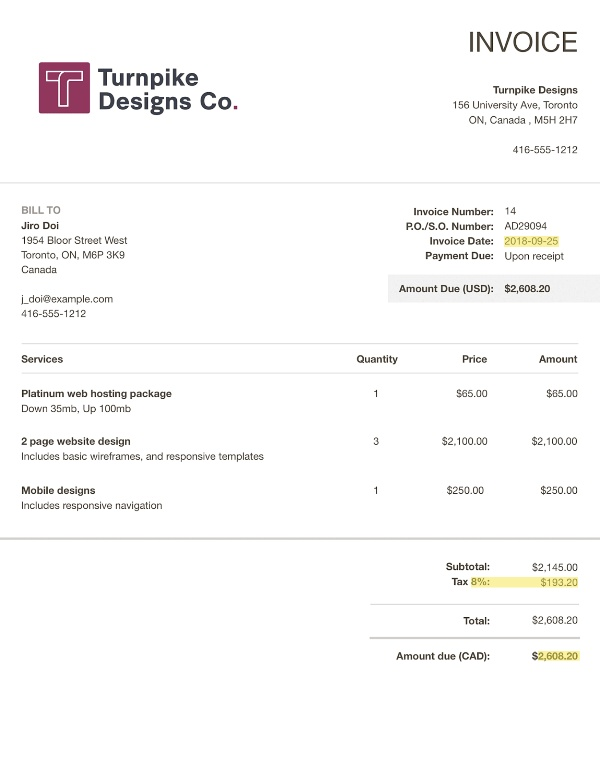## Conclusion

In just over a few seconds, an invoice was parsed and the features were highlighted.

If you wanted to use this kind of script to display results to your users, i'd advise you to do the highlighting in the front-end application, as sending images back from your middleware is not the best option because of payload sizes. The other solution would be to store the final image using cv2.imwrite(...) but it would make your client download the result.

If you have questions, please reach out to us in the chat widget in the bottom right.